#### Vector fields as velocity fields

We now give a particular interpretation to vector fields. A vector field expressed using (8.10) can be used to define a set of first-order differential equations as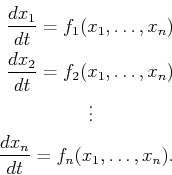(8.11)

Each equation represents the derivative of one coordinate with respect to time. For any point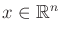, a velocity vector is defined as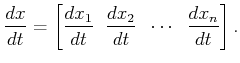(8.12)

This enables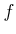to be interpreted as a velocity field.

It is customary to use the short notation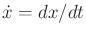. Each velocity component can be shortened to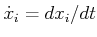. Usingto denote the vector of functions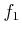,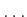,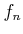, (8.11) can be shorted to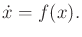(8.13)

The use ofhere is an intentional coincidence with the use offor the state transition equation. In Part IV, we will allow vector fields to be parameterized by actions. This leads to a continuous-time state transition equation that looks like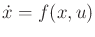and is very similar to the transition equations defined over discrete stages in Chapter 2.

The differential equations expressed in (8.11) are often referred to as autonomous or stationary becausedoes not depend on time. A time-varying vector field could alternatively be defined, which yields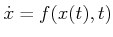. This will not be covered, however, in this chapter.

Steven M LaValle 2020-08-14